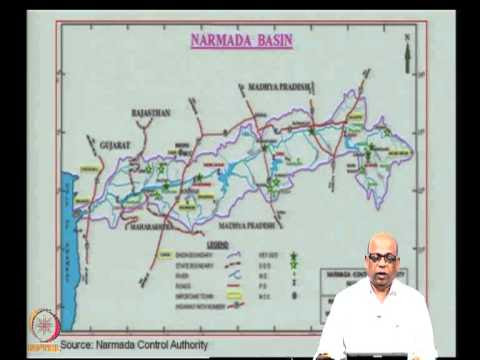### Water Resources Systems : Modeling Techniques and AnalysisCourse Description: The course introduces the concepts of systems techniques in water resources planning and management.
Course Contents
Introduction – Concepts of Systems and Systems Analysis; Systems Techniques in Water Resources : Optimization with methods using calculus; Linear Programming; Dynamic Programming; Simulation; Combination of Simulation and Optimzation; Mutli-objective Planning. Economic Considerations in Water Resources Planning; Reservoir Systems – Deterministic Inflow : Reservoir Sizing; Reservoir Operation – standard operating policy, optimal operating policy; multi-reservoir systems; Reservoir Systems – Random Inflow : Chance Constrained Linear Programming; Concept of Reliability; Stochastic Dynamic Programming; Applications – Reservoir systems operated for Irrigation, Hydropower, Flood Control and Municipal and Industrial Supplies; Water Quality Control in River Stystems; Case Studies; Recent Modeling Tools – Artificial Neural networks, Fuzzy Inference Systems; Fuzzy Linear Programming;
 Module 1 Introduction and Optimization Lecture-1 Introduction Lecture-2 Definitions and types of systems Lecture-3 Optimization: Functions of a single variable Lecture-4 Optimization: Functions of multiple variables Lecture-5 Constrained optimization (1) Lecture-6 Constrained optimization (2) Lecture-7 Kuhn-Tucker conditions and Introduction to Linear Programming Module 2 Linear Programming Lecture-8 Linear Programming: Graphical method Lecture-9 Linear Programming: Simplex method (1) Lecture-10 Linear Programming: Simplex method (2) Lecture-11 Linear Programming: Multiple solutions Lecture-12 Linear Programming: Unbounded and infeasible problems Lecture-13 Linear Programming: Dual problem Module 3 Dynamic Programming Lecture-14 Introduction to Dynamic Programming Lecture-15 Dynamic Programming: Water allocation problem Lecture-16 Dynamic Programming: Reservoir operation problem Lecture-17 Dynamic Programming: Capacity expansion and shortest route problems Module 4 Simulation and Multi-Objective Planning Lecture-18 Simulation: Introduction to Multi-objective planning Lecture-19 Multi-objective planning Module 5 Reservoir Systems – Deterministic inflows Lecture-20 Reservoir sizing Lecture-21 Reservoir capacity using Linear Programming (1) Lecture-22 Reservoir capacity using Linear Programming (2) Lecture-23 Reservoir operation Lecture-24 Multi-reservoir systems Lecture-25 Stationary policy using Dynamic Programming Lecture-26 Hydropower generation Module 6 Reservoir Systems – Random inflows Lecture-27 Basic probability theory (1) Lecture-28 Basic probability theory (2) Lecture-29 Chance constrained Linear Programming for reservoir operation and design (1) Lecture-30 Chance constrained Linear Programming for reservoir operation and design (2) Lecture-31 Stochastic Dynamic Programming for reservoir operation (1) Lecture-32 Stochastic Dynamic Programming for reservoir operation (2) Lecture-33 Stochastic Dynamic Programming for reservoir operation (3) Module 7 Fuzzy Optimization Lecture-34 Fuzzy optimization (1) Lecture-35 Fuzzy optimization (2) Lecture-36 Fuzzy optimization for water quality control and reservoir operation Module 8 Model Formulations and Case Studies Lecture-37 Conjunctive use of ground and surface water Lecture-38 Hydropower optimization Lecture-39 Crop yield optimization Lecture-40 Multi-basin and multi-reservoir systems